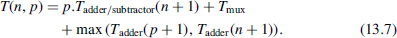## 13.2 INTEGERS

### 13.2.1 Base-2 Nonrestoring Divider

Let Y be an n-bit positive number and X an integer belonging to the range − Y ≤ X < Y, so that it can be represented as an (n + 1)-bit 2's complement number. The circuit corresponding to the nonrestoring algorithm 6.6 (with q(i) substituted by q(p − i) in order that the least significant bit of q be q(0)) is shown in Figures 13.6 (basic cell), 13.7 (divider structure, combinational and sequential implementations), and 13.8 (correction circuit).

The cost and computation time of the corresponding divider areandExamples 13.4 (Complete VHDL source code available.) Generate a VHDL model of a generic base-2 nonrestoring divider (Figures 13.6, 13.7, and 13.8):

```entity nonr_cell is
port (
a: in STD_LOGIC_VECTOR (N-1 downto 0);
b: in STD_LOGIC_VECTOR (N-1 downto 0);
q: in STD_LOGIC;
r: out STD_LOGIC_VECTOR (N downto 0)
);
end nonr_cell;

architecture nr_cel_arch of nonr_cell is
signal a_by_2: STD_LOGIC_VECTOR (N downto 0);
begin
a_by_2<=a(N-1 downto 0)&‘0’;
begin
if q=‘1’ then r<=a_by_2+b;
else r<=a_by_2 - b;
end if;
end process;
end nr_cel_arch;```Figure 13.6 Nonrestoring divider: basic cell.

Figure 13.7 ...

Get Synthesis of Arithmetic Circuits: FPGA, ASIC and Embedded Systems now with the O’Reilly learning platform.

O’Reilly members experience books, live events, courses curated by job role, and more from O’Reilly and nearly 200 top publishers.